10 October 2015

# Week 3: Initial Predictions

## Dimensionality Reduction Process

#### t-SNE Review

Approximation algorithm only relies on distance vectors, has linearithmic time and linear space requirements. Only downside is that it’s a black box, so we can’t update our dimensionality reduction based on the results of our predictions.

To give a brief glance into the paper (see last post for the link), here’s the definition of the “similarity distribution” in high-dimensional space.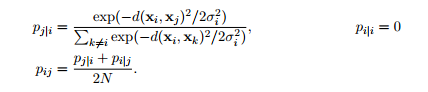As you can expect, the KL divergence, which we’re trying to optimize, will have a quadratic number of terms since there’s $$O(N^2)$$ such $$p_{ij}$$.

Thus, for faster computation, we need a smaller approximate representation, which relies on neighborhoods constructed from nearest neighbors $$\mathcal{N}_i$$.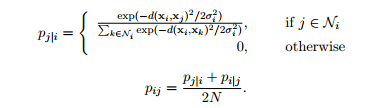Note for completeness the low-dimensional similarity is given by: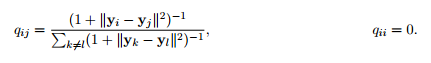This is the exact form of the low-dimensional Student-t distribution. We never really compute it explicitly, since we don’t actually care what the low-dimensionality similarity distribution $$Q$$ is, but rather only are concerned with the representation of the KL divergence - or, more precisely, its gradient, which we are optimizing with the projected values $$\textbf{y}_i$$.

The quadratic term in the gradient is interestingly similar enough to an $$n$$-body simulation problem that we can use the Barnes-Hut approximation problem to approximate it.

Note the above step implies the use of a $$2^d$$-tree, where $$d$$ is the dimension we’re reducing to, so there’s a serious cap on $$d$$.

#### t-SNE Results

MNIST

Double-encoded MNIST (from paper, had PCA run initially to 50 dims for speed):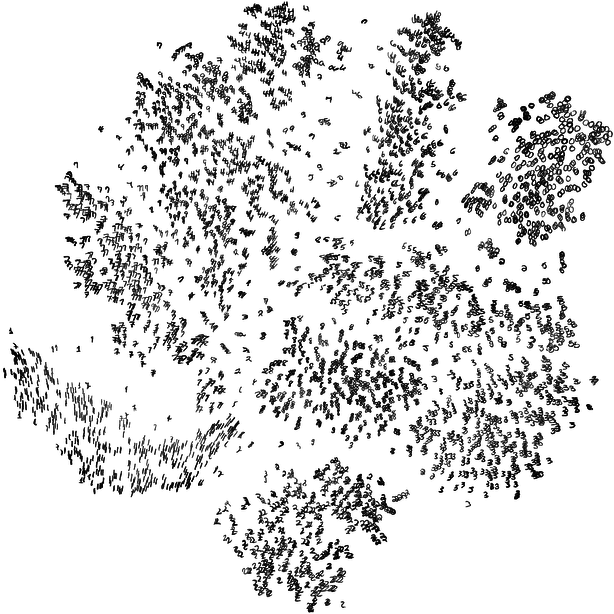Byte-encoded MNIST (t-SNE only in my fork), 10000 imgs x (28x28) pixels trains in 164s: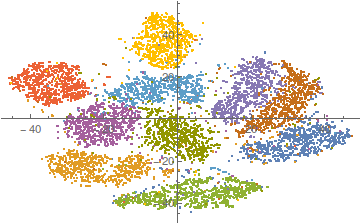GDELT

News data reduction. 2 days’ worth of data - 326604 x 14 data matrix, with categories. Took <2 hrs. Just two instantiations for parameters, perplexity $$u = 30$$ for the high-dimensional distribution and cutoff $$\theta = 0.5$$ were used. Note the higher default perplexity causes the typical set to be larger, which in turn results in high standard deviations in the high-dimensional distribution. When encoded to the low-dimensional distribution, this means that all the points look like they’re all just as similar to one another (because the high-dimensional kernels all look the same). In turn we have a tendency towards equidistance in the low dimensions, forcing the data to aggregate in a circular blob.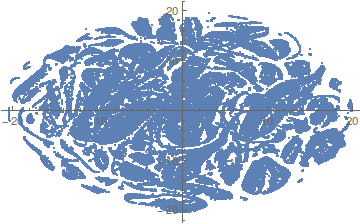Nonetheless, there are some definitive clusters already. The extracted columns used were (with additional preprocessing):

#### HMM Results

Clustering data points into: up trend / down trend Feeding only the actual prices to the HMM is not sufficient, because the trends are not very clear in commodities (by opposition to stocks for example) => erratic predictions. We remedy to this problem by adding the moving average as input so that the model is more stable.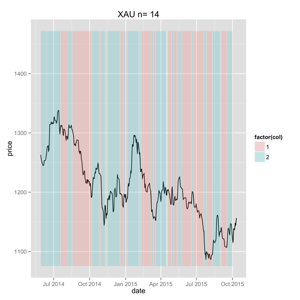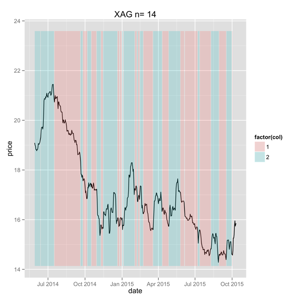Train data in different clusters independently using a linear model.